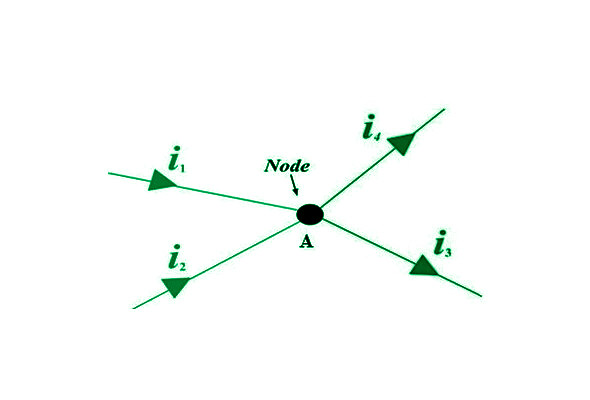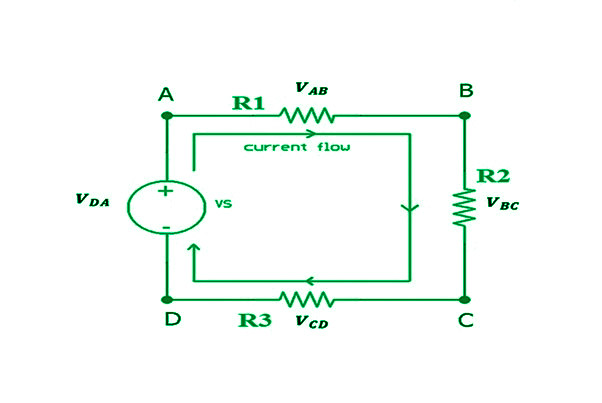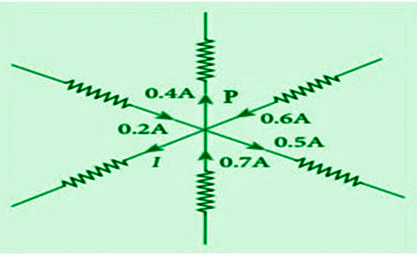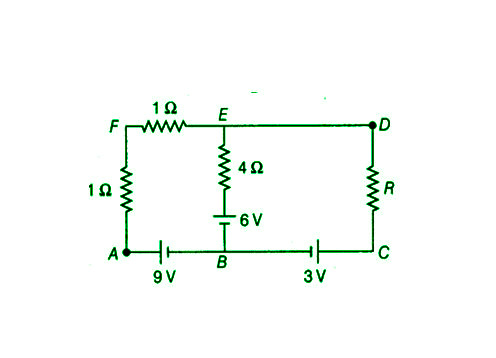Kirchoff’s Circuit Law

• Last Updated : 29 Oct, 2021

A rush of charged particles, such as electrons or particles, travelling across an electrical channel or area is known as an electric flow. It’s calculated as the net speed of an electric charge stream passing through a surface or into a control volume. Charge transporters are the moving particles, and depending on the transmitter, they might be one of several types of particles. Electrons travelling over a wire are commonly used as charge transporters in electric circuits.

They can be electrons or openings in semiconductors. The charge carriers in an electrolyte are particles, whereas they are particles and electrons in the plasma, an ionised gas. The ampere, or amp, is the SI unit of electric flow, which is defined as the passage of electric charge over a surface at a rate of one coulomb per second. The ampere is a standard SI unit of measurement. An ammeter is used to calculate the amount of electricity flowing through a circuit. Engines, generators, inductors, and transformers all use attractive fields created by electric fluxes. They generate Joule warming in conventional conductors, which produces light in glowing lights. Electromagnetic waves are produced by time-varying fluxes and are used in media communications to transmit data.

Kirchhoff’s Law

Gustav Robert Kirchhoff was a Russian-born German physicist. His job was investigating electrical conduction. Kirchhoff’s Voltage Law (KVL) and Kirchhoff’s Current Law (KCL) were published in 1845. (KCL). Kirchhoff’s Circuit Law is the result of their combination. Circuit analysis is carried out using these laws. They aid in the calculation of current flow in various streams across the network.

• Kirchhoff’s First Law: This law says that “the total current or charge entering a junction or node is precisely identical to the total current or charge exiting the node, as no charge is lost at the node.” To put it another way, the algebraic total of all currents entering and exiting a node must be zero. It is also known as Kirchhoff’s Current Law (KCL).

Note: Kirchhoff’s current law is in favour of charge conservation. As a result, a Nord or junction is a point in a circuit that does not serve as a charge source or sink.

Therefore,

nk=1 IK = 0

Where n denotes the total number of branches at the node with currents flowing toward or away from it.

i.e.

I(exiting)+I(entering) = 0

For Example,

From a resistor network, concentrate on node A. This node is related to four branches. Two incoming currents, i1 and i2, and two outgoing currents, i3 and i4, are present. The sum of total incoming and outgoing currents at node A will now equal zero, according to Kirchhoff’s current law.Consider the two currents that enter the node, i1 and i2, as positive, and the two currents that leave the node, i3 and i4, as negative.

i1+i2–i3–i4=0

• Kirchhoff’s Second Law: The voltage drop around a loop equals the algebraic total of the voltage drop across every electrical component linked in the same loop for any closed network and also equals zero, it is also known as Kirchhoff’s Voltage Law (KVL).

Notes: Kirchhoff’s Voltage Rule is based on the law of conservation of energy. Because the net change in the energy of a charge after it completes a closed route must be zero.

For Example,

Consider a section of a resistor network with an internal closed-loop, as seen in the diagram. In the closed-loop, we wish to write the voltage decreases. The total of all voltage drops between the components linked in the loop ABCDA is zero, according to Kirchhoff’s voltage law.So,

nk=1 Vk=0

The total number of electrical components in the loop is given by n.

i.e.

VAB+VBC+VCD+VDA=0

Applying Kirchhoff’s Laws

When using KCL, the currents exiting a junction must be considered negative, while the currents entering the junction must be considered positive.

Also, while using KVL, we keep the same anti-clockwise or clockwise orientation from the beginning of the loop and account for all voltage decreases as negative and increases as positive. This brings us back to the initial point when the total voltage loss is zero.

Sign conventions:

1. In a loop, a rise in potential difference or EMF from lower to higher is always seen as positive.
2. In a loop, a reduction in potential difference or EMF from higher to lower is always seen as negative.
3. If the looping direction is the same as the current flowing through the circuit, the voltage drop across the resistor is considered negative.

Uses of Kirchhoff’s Law

• It is used to find out how much current is flowing and how much voltage is dropped in various areas of the complicated circuit.
• It aids in determining the direction of current in various circuit loops.
• Kirchhoff’s Laws can help you comprehend how energy moves via an electric circuit.

Kirchhoff’s Laws Limitations

1. Kirchhoff’s law has one fundamental flaw: it implies that there is no changing magnetic field throughout the loop’s region, which might induce a change in magnetic flux and the creation of EMF in the circuit. This might result in a calculation mistake for high-frequency AC circuits.
2. Kirchhoff also ignored the impact of the electric field generated by other circuit components.
3. KCL works with the premise that current only travels through conductors and wires. While parasitic capacitance can no longer be overlooked in High-Frequency circuits. Because conductors or wires are serving as transmission lines in certain circumstances, electricity may start flowing in an open circuit.

Sample Questions

Question 1: What is the rule of junction and loop?

The junction rule, also known as Kirchhoff’s Current Law (KCL), states that at any given junction, the sum of the entering currents equals the sum of the outgoing currents.

Kirchhoff’s Loop Rule, often known as Kirchhoff’s Voltage Law, states that the sum of the voltage differences around the loop must equal zero (KVL).

Question 2: What is the definition of Node Voltage?

We’re referring about the difference in potential between two nodes in a circuit when we say node voltage. One of the nodes in the circuit is chosen as a reference node. The voltages of all other nodes are measured in respect to this single reference node.

Question 3: Find the value of I from the circuit in the figure below.Apply Kirchhoff’s first law to the point P in the supplied circuit.

Consider the sign convention: positive arrows point toward P, whereas negative arrows point away from P.

As a result, we now have the following:

0.2 A – 0.4 A + 0.6 A – 0.5 A + 0.7 A – I = 0

1.5 A – 0.9 A – I = 0

0.6 A – I = 0

I = 0.6A

Question 4: What significance does Kirchhoff’s law have?

Kirchhoff’s principles can be used to calculate the values of unknown circuit variables like current and voltage. These principles may be applied to any circuit and can be used to identify unknown values in intricate circuits and networks (with certain limits). It assists in the comprehension of energy transfer in various circuit components.

Question 5: Find the value of unknown resistance R in the circuit below using Kirchhoff’s principles, ensuring that no current flows through the 4 ohms resistance. Find the possible difference between points A and D as well.Because there is no current passing through the 4 resistor , all current going along FE will travel through ED (per Kirchhoff’s first law).

Now, in mesh AFEBA, using Kirchhoff’s second law,

We have:- –1 × I – 1 × I – 4 × 0 – 6 + 9 = 0

–2I + 3 = 0

I = 3/2 A                                                                                                                                         –(1)

Using Kirchhoff’s 2nd rule in mesh AFDCA once again,

We have: –1×I–1×I–I×R–3+9=0

–2I–IR+6=0

2I+IR=6      –(2)

From equations (1) and (2), we get

(2×3/2)+3/2xR=6

R=2Ω

For probable variations between A and D, as well as AFD,

We have:- VA – 3/2×1 – 3/2×1=VD

VA–VD=3V

Question 6: Why does Kirchhoff’s law fail at high frequency?